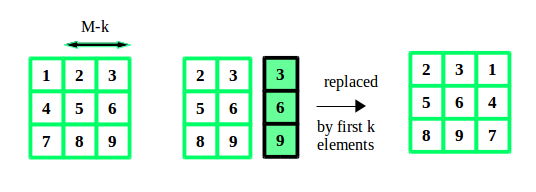# Java Program for Rotate the matrix right by K times

• Last Updated : 08 Jun, 2022

Given a matrix of size N*M, and a number K. We have to rotate the matrix K times to the right side.
Examples:

```Input :  N = 3, M = 3, K = 2
12 23 34
45 56 67
78 89 91

Output : 23 34 12
56 67 45
89 91 78

Input :  N = 2, M = 2, K = 2
1 2
3 4

Output : 1 2
3 4```

A simple yet effective approach is to consider each row of the matrix as an array and perform an array rotation. This can be done by copying the elements from K to end of array to starting of array using temporary array. And then the remaining elements from start to K-1 to end of the array.
Lets take an example:## Java

 `// Java program to rotate a matrix``// right by k times` `class` `GFG``{``    ``// size of matrix``    ``static` `final` `int` `M=``3``;``    ``static` `final` `int` `N=``3``;``    ` `    ``// function to rotate matrix by k times``    ``static` `void` `rotateMatrix(``int` `matrix[][], ``int` `k)``    ``{``        ``// temporary array of size M``        ``int` `temp[]=``new` `int``[M];``        ` `        ``// within the size of matrix``        ``k = k % M;``        ` `        ``for` `(``int` `i = ``0``; i < N; i++)``        ``{``        ` `            ``// copy first M-k elements``            ``// to temporary array``            ``for` `(``int` `t = ``0``; t < M - k; t++)``            ``temp[t] = matrix[i][t];``        ` `            ``// copy the elements from k``            ``// to end to starting``            ``for` `(``int` `j = M - k; j < M; j++)``            ``matrix[i][j - M + k] = matrix[i][j];``        ` `            ``// copy elements from``            ``// temporary array to end``            ``for` `(``int` `j = k; j < M; j++)``            ``matrix[i][j] = temp[j - k];``        ``}``    ``}``    ` `    ``// function to display the matrix``    ``static` `void` `displayMatrix(``int` `matrix[][])``    ``{``        ``for` `(``int` `i = ``0``; i < N; i++)``        ``{``            ``for` `(``int` `j = ``0``; j < M; j++)``            ``System.out.print(matrix[i][j] + ``" "``);``            ``System.out.println();``        ``}``    ``}``    ` `    ``// Driver code``    ``public` `static` `void` `main (String[] args)``    ``{``        ``int` `matrix[][] = {{``12``, ``23``, ``34``},``                        ``{``45``, ``56``, ``67``},``                        ``{``78``, ``89``, ``91``}};``    ``int` `k = ``2``;``    ` `    ``// rotate matrix by k``    ``rotateMatrix(matrix, k);``    ` `    ``// display rotated matrix``    ``displayMatrix(matrix);``    ``}``}` `// This code is contributed by Anant Agarwal.`

Output:

```23 34 12
56 67 45
89 91 78```

Time Complexity: O(N*M)
Auxiliary Space: O(M)

Please refer complete article on Rotate the matrix right by K times for more details!

My Personal Notes arrow_drop_up